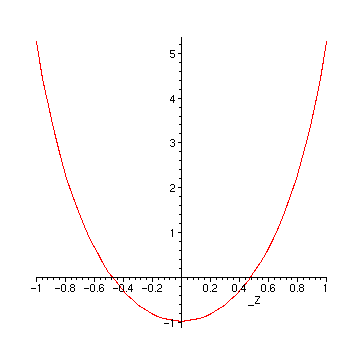Finding Extrema of a Function

We want to find the extrema of the function

> f := sin(x)*exp(-2*x^2);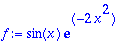> df := diff(f, x);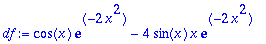> solve(%, x);Since maple can't solve this equation analytically, we ask for a numeric solution:

> fsolve(df, x);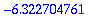We don't trust this simple solution blindly, but try to get a qualitative overview by plotting the function:

> plot(f, x=-10..10);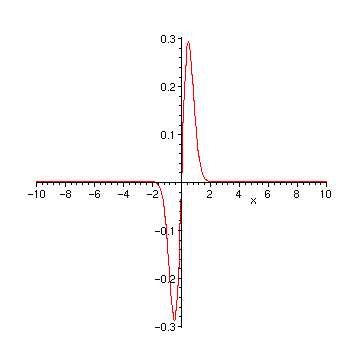This doesn't seem to agree with the numeric result, so we take a closer look:

> plot(df, x=-6.5..-6);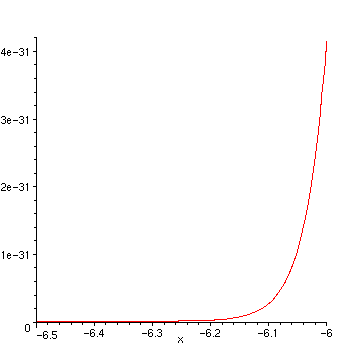The high precision of maple calculations allows us to refine even more:

> plot(f, x=-6.4..-6.3);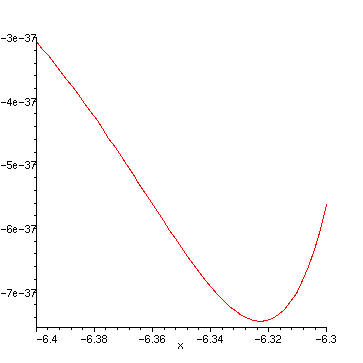This at last confirms the former result. But we are more interested in the "main" peak between 0 and 1:

> fsolve(df, x, 0..1);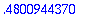And again we use a plot for a gross check of the numerics:

> plot(df, x=0..1);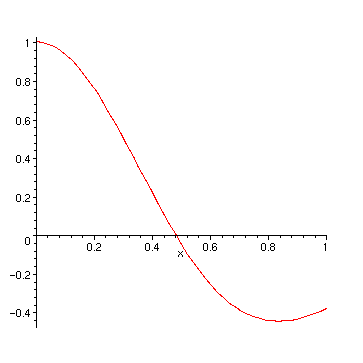To get an overview of all solutions, we go back to the algebraic solution:

> minmax := solve(df, x);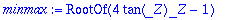> sol := op(1,%);A direct plot of this equation doesn't help much:

> plot(sol, _Z=-10..10);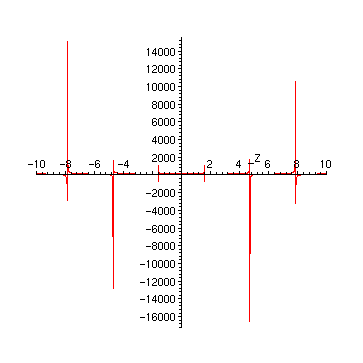The reason for the strange plot are of course the poles of the tan. A first improvement is to restrict the range values:

> plot(sol, _Z=-10..10, -100..100);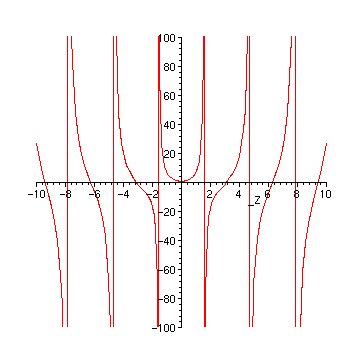Finally, the nasty lines at the poles disappear, if we plot only the continuous parts:

> plot(sol, _Z=-10..10, -100..100, discont=true, color=red);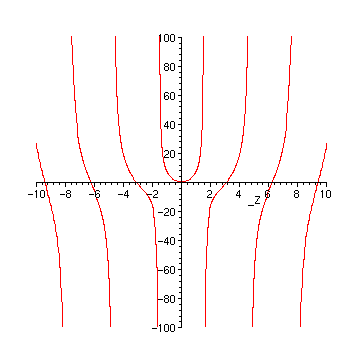Only the region around 0 needs a closer look:

> plot(sol, _Z=-1..1);